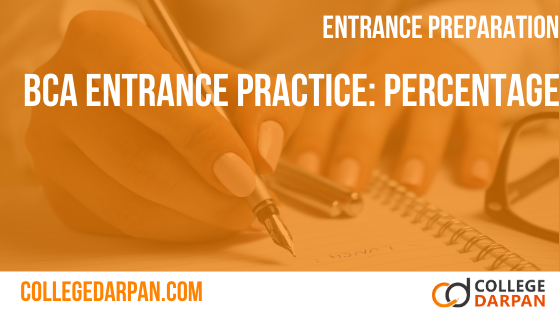# BCA Entrance Practice Quiz: Percentage

1. What will be the fraction of 4%
2. 3.05 can be expressed in terms of percentage as
3. What is 15 percent of 34
4. 2 is what percent of 50
5. What percent is 250 gram of 1 Kg?
6. What percent is 25 paisa of Rs. 10?
7. 25% of a number is 300. The number is?
8. A primary school had an enrollment of 850 pupils in January 1970. In January 1980 the enrollment was 1120. What was the percentage increase for the enrollment?
9. In a co-education secondary school 55% of the students are boys. There are 360 girls in the school. The number of boys in the school is?
10. A student has to secure 40% marks to pass. He gets 40 marks and fails by 40 marks. The maximum number of marks is?
11. After spending 88% of his income, a man had Rs. 2160 left. Find his income.
12. In an examination, 900 students were boys and 1100 were girls. If 32% of the boys and 38% of the girls passed the examination, the total percentage (of boys and girls together) of candidates that failed will be?
13. In an examination, 900 students were boys and 1100 were girls. If 32% of the boys and 38% of the girls passed the examination, the total percentage (of boys and girls together) of candidates that failed will be?
14. If 40 students sit for an aptitude test and 8 of them failed, what percentage of them passed the test?
15. A man's new income is Rs. 8400 per month. Given that his original income was Rs. 7500 per month, find the percentage increase in his monthly income
16. A tour guide earns commission by bringing tourists to patronise a certain handicraft shop. Given that the commission he receives is 3% of the total sales. Calculate his commission on a particular day when the 12 tourists he brought to the shop spent an average of Rs. 2500 each.
17. If 10% is deducted from a bill, Rs. 585 remains to be paid. How much is the bill?
18. If 10% is deducted from a bill, Rs. 585 remains to be paid. How much is the bill?
19. The enrollment in a certain secondary school was 450 in 1979. By 1980 the enrollment had increased by 16%. What was the enrollment in 1980?
20. A fruit seller has 120 oranges. Given that he has 20% more apples than oranges and 40% less oranges than pears, find the number of apples and the number of pears the fruit seller has?
21. A boy scored 90 marks for his mathematics test. This was 20% more than what he had scored for the geography test. How much did he score in geography?
22. In an examination a candidate has to secure 40% marks to pass. If a candidate secures 190 marks and fails by 10 marks. The total number of marks in the examination is?
23. If 50% of y is 250, then y is 50% of
24. Three teams scored in the tournament as 125, 375 and 250 respectively. What percentage of the total score did the winning team get?
25. In an examination 33% of the students failed in Mathematics, 27% failed in English, while 15% of the students failed in both the subjects. Find the percentage of the students, who passed in both the subjects.
26. Two students appeared at an examination. One of them secured 9 marks more than the other and his marks was 56% of the sum of their marks. The marks obtained by them are
27. After deducting 20% from a certain sum and then 30% from the remainder, there is 3500 left. Find the original sum?
28. If A's salary is 35% less that of B, then how much percent is B's salary less than that of A?
29. If there are 50 students in class and 95% got passed then the students who failed the test (in numbers) are
30. If 200 students filled the form for entrance test and 180 appeared in the test out of which only 70% have passed the test then the number of students who failed the test are
31. In a shoe factory, 2 2∕5 % shoes made are defective. If the company made 10,000 shoes then the number of defective pieces are
32. In math's test, Hazel scored 92% marks out of 150. Marks obtained by Hazel are
33. A’s salary is 50% more than B’s. How much percent is B’s salary less than A’s?
34. The population of a town increases 20% annually. If it’s present population is 1,20,000. Find its population after 3 years?
35. If population of Meerut City is 360. If population of Meerut City grows by 22.5 % in a year. Calculate the population after one year.
36. The population of a town increased from 2,00,000 to 2,80,000 in a decade. What is the increase in percentage?